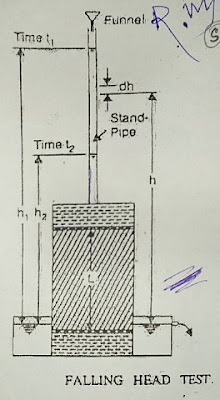Determine Coefficient of Permeability of Soil by Falling Head Permeameter - Civil Engineering

Co-efficient of Permeability by Falling Head Permeameter

This test is suitable for fine-grained soils.
The apparatus used for this test is called falling head permeameter.Coefficient of Permeability of Soil by Falling Head Permeameter
The figure shows the diagrammatic representation of this test.
The soil sample is kept in a vertical cylinder of cross-sectional area A and length L. A transparent standpipe sectional area 'a' are attached to the test cylinder.

The test cylinder is kept in a container filled with water of constant level. the soil sample is saturated by allowing the water to flow through the sample continuously. After the saturation is complete, the standpipe is filled with water and the water is allowed to run down and a stopwatch is started.

The head at any time instant 't' is equal to the difference in the water level in the standpipe and the bottom tank. Let h1 and h2 be the heads at time interval t1 and t2 (t2>t1)respectively.

Let 'h' be the head at any intermediate time interval 't' and dh be the change in the head in 'a' smaller time interval dt. Hence, from Darcy's law, the rate of flow q is given by.
q = (- dh.a )/dt = K.i.A
[minus sign has been used since 'h' decrease as 't' increase]
where m i = Hydraulic gradient at time 't' = h/L

K.h.A/L = -dh.a /dt
or, A.K.dt/a.L = -dh/h
Integrating between two-time limits, we getCoefficient of Permeability of Soil